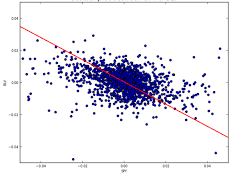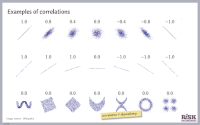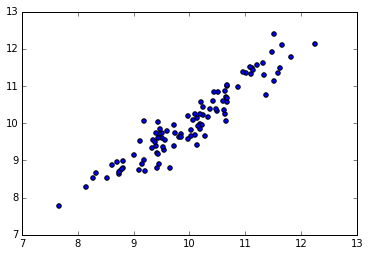# Correlation analysis How are my variables related?

## OverviewBefore you start to create risk models, it is a good idea to visualize how the variables are related to one another. Risk models often involve correlated random variables, and exploring correlation between variables is an important part of exploratory data analysis. Learn how to measure the degree of linear dependency between two random variables using Python and the SciPy library. The lecture slides include a number of warnings related to dependency, causality and correlation in risk analysis.

This submodule is a part of the risk analysis module.

## Course materialModelling correlations with PythonLecture slides (PDF)Python notebook on correlationDownload Python notebookRun notebook in MyBinderRun notebook in Google ColabIn these course materials, applications are presented using the NumPy, SciPy and statsmodels libraries for the Python programming language. We have some material on getting started with Python that explains how to install Python on your computer or try out our computational notebooks using free online services.

## Other resources

We recommend the following sources of further information on this topic:

Published: